Courses

# Graphs MCQ - 1

## 20 Questions MCQ Test RRB JE for Computer Science Engineering | Graphs MCQ - 1

Description
This mock test of Graphs MCQ - 1 for Computer Science Engineering (CSE) helps you for every Computer Science Engineering (CSE) entrance exam. This contains 20 Multiple Choice Questions for Computer Science Engineering (CSE) Graphs MCQ - 1 (mcq) to study with solutions a complete question bank. The solved questions answers in this Graphs MCQ - 1 quiz give you a good mix of easy questions and tough questions. Computer Science Engineering (CSE) students definitely take this Graphs MCQ - 1 exercise for a better result in the exam. You can find other Graphs MCQ - 1 extra questions, long questions & short questions for Computer Science Engineering (CSE) on EduRev as well by searching above.
QUESTION: 1

Solution:
QUESTION: 2

Solution:
QUESTION: 3

### The time complexity of computing the transitive closure of a binary relation on a set of n elements is known to be:

Solution:
QUESTION: 4

The most efficient algorithm for finding the number of connected components in an undirected graph on n vertices and m edges has time complexity.

Solution:

Connected components can be found in O(m + n) using Tarjan's algorithm. Once we have connected components, we can count them.

QUESTION: 5

Consider an undirected unweighted graph G. Let a breadth-first traversal of G be done starting from a node r. Let d(r, u) and d(r, v) be the lengths of the shortest paths from r to u and v respectively, in G. lf u is visited before v during the breadth-first traversal, which of the following statements is correct? (GATE CS 2001)

Solution:

d(r, u) and d(r, v) will be equal when u and v are at same level, otherwise d(r, u) will be less than d(r, v)

QUESTION: 6

How many undirected graphs (not necessarily connected) can be constructed out of a given set V= {V 1, V 2,…V n} of n vertices ?

Solution:

In an undirected graph, there can be maximum n(n-1)/2 edges. We can choose to have (or not have) any of the n(n-1)/2 edges. So, total number of undirected graphs with n vertices is 2(n(n-1)/2).

QUESTION: 7

Which of the following statements is/are TRUE for an undirected graph?
P: Number of odd degree vertices is even
Q: Sum of degrees of all vertices is eve

Solution:

P is true for undirected graph as adding an edge always increases degree of two vertices by 1. Q is true: If we consider sum of degrees and subtract all even degrees, we get an even number because every edge increases the sum of degrees by 2. So total number of odd degree vertices must be even.

QUESTION: 8

Consider an undirected random graph of eight vertices. The probability that there is an edge between a pair of vertices is 1/2. What is the expected number of unordered cycles of length three?

Solution:

A cycle of length 3 can be formed with 3 vertices. There can be total 8C3 ways to pick 3 vertices from 8. The probability that there is an edge between two vertices is 1/2. So expected number of unordered cycles of length 3 = (8C3)*(1/2)3 = 7

QUESTION: 9

Given an undirected graph G with V vertices and E edges, the sum of the degrees of all vertices is

Solution:

Since the given graph is undirected, every edge contributes as 2 to sum of degrees. So the sum of degrees is 2E.

QUESTION: 10

What is the maximum number of edges in an acyclic undirected graph with n vertices?

Solution:

n * (n - 1) / 2 when not cyclic. An acyclic graph with the maximum number of edges is actually a spanning tree and therefore, correct answer is n-1 edges. So Answer is A

QUESTION: 11

Let G be a weighted undirected graph and e be an edge with maximum weight in G. Suppose there is a minimum weight spanning tree in G containing the edge e. Which of the following statements is always TRUE?

Solution:

Background : Given a connected and undirected graph, a spanning tree of that graph is a subgraph that is a tree and connects all the vertices together.

1. A spanning tree has exactly V - 1 edges.
2. A single graph can have many different spanning trees. A minimum spanning tree (MST) or minimum weight spanning tree for a weighted, connected and undirected graph is a spanning tree with weight less than or equal to the weight of every other spanning tree. The weight of a spanning tree is the sum of weights given to each edge of the spanning tree.
3. There can be more that one possible spanning trees of a graph. For example, the graph in this question has 6 possible spanning trees.
4. MST has lightest edge of every cutset. Remember Prim's algorithm which basically picks the lightest edge from every cutset.

Choices of this question : a) There exists a cutset in G having all edges of maximum weight : This is correct. If there is a heaviest edge in MST, then there exist a cut with all edges with weight equal to heavies edge. See point 4 discussed in above background.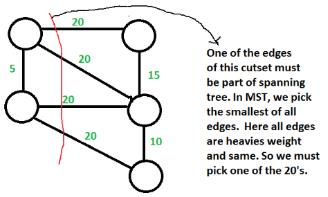b) There exists a cycle in G having all edges of maximum weight : Not always TRUE. The cutset of heaviest edge may contain only one edge. In fact there may be overall one edge of heavies weight which is part of MST (Consider a graph with two components which are connected by only one edge and this edge is the heavies) c) Edge e cannot be contained in a cycle. Not Always True. The curset may form cycle with other edges. d) All edges in G have the same weight Not always True. As discussed in option b, there can be only one edge of heaviest weight.

QUESTION: 12

A sink in a directed graph is a vertex i such that there is an edge from every vertex j ≠ i to i and there is no edge from i to any other vertex. A directed graph G with n vertices is represented by its adjacency matrix A, where A[i] [j] = 1 if there is an edge directed from vertex i to j and 0 otherwise. The following algorithm determines whether there is a sink in the graph G.

i = 0
do {
j = i + 1;
while ((j < n) && E1) j++;
if (j < n) E2;
} while (j < n);
flag = 1;
for (j = 0; j < n; j++)
if ((j! = i) && E3)
flag = 0;
if (flag)
printf("Sink exists");
else
printf("Sink does not exist");

Q. Choose the correct expressions for E3

Solution:

Below explanation is for Previous Part of this question: For vertex i to be a sink, there should be no edge from i to any other vertex.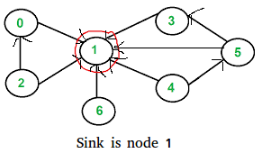According the input given in question,

A[i][j] = 1 means there is an edge from vertex i to j.
A[i][j] = 0 means there is no edge from i to j

For a node to i to be sink,

A[i][j] should be 0 for all j
A[j][i] should be 1 for all j.

The above pseudo code checks every vertex i for sink, starting from i = 0. It basically checks every vertex j after i for being a sink. The trick in pseudo code is, it doesn't check for j smaller than i. The i picked by this loop may not be sink. It basically makes sure that we don't ignore a potential sink. The check whether i is actually a sink or not is done later after do while loop. Vertex i is a potential sink while A[i][j] is zero Thus, E1 : !A[i][j] If the above condition is false, then i is not a sink. All j < i can also not be a sink because there is no edge from i to j. Now, the next potential sink can be j. So, E2 : i = jExplanation for this question The following pseudo code basically checks if the potential sink picked by the code above is actually a sink or not.

flag = 1;
for (j = 0; j < n; j++)
if ((j! = i) && E3)
flag = 0;

flag equals to 0 means i is not a sink. The code sets the flag 0 as soon as it finds out that i is not a sink.

A node i is not a sink if either of the following two conditions become true for any j not equal to i.
A[i][j] is 1 for any j
OR A[j][i] is 0 for any j

E3 : (A[i][j] | | !A[j][i]) Therefore option D is correct

QUESTION: 13

Consider a simple graph with unit edge costs. Each node in the graph represents a router. Each node maintains a routing table indicating the next hop router to be used to relay a packet to its destination and the cost of the path to the destination through that router. Initially, the routing table is empty. The routing table is synchronously updated as follows. In each updation interval, three tasks are performed.

1. A node determines whether its neighbours in the graph are accessible. If so, it sets the tentative cost to each accessible neighbour as 1. Otherwise, the cost is set to ∞.
2. From each accessible neighbour, it gets the costs to relay to other nodes via that neighbour (as the next hop).
3. Each node updates its routing table based on the information received in the previous two steps by choosing the minimum cost.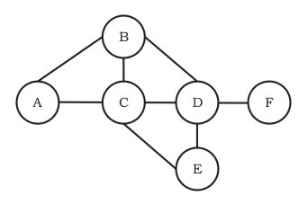For the graph given above, possible routing tables for various nodes after they have stabilized, are shown in the following options. Identify the correct table.

Solution: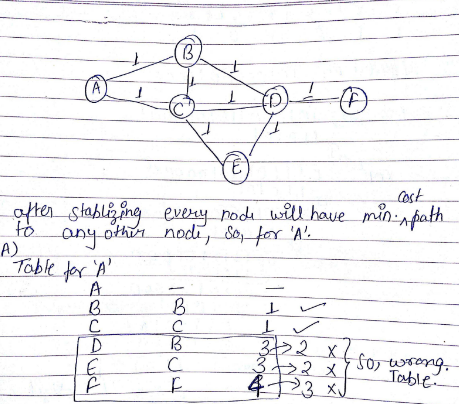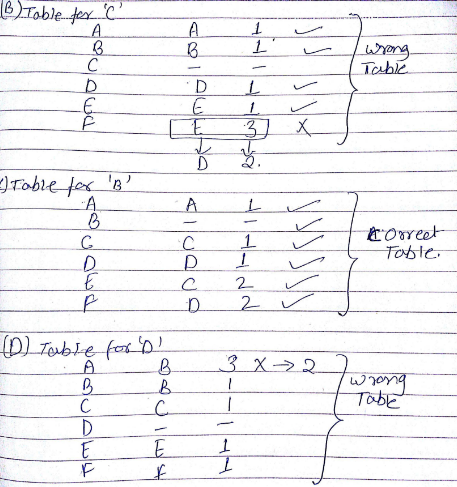QUESTION: 14

Consider a simple graph with unit edge costs. Each node in the graph represents a router. Each node maintains a routing table indicating the next hop router to be used to relay a packet to its destination and the cost of the path to the destination through that router. Initially, the routing table is empty. The routing table is synchronously updated as follows. In each updation interval, three tasks are performed.

1. A node determines whether its neighbours in the graph are accessible. If so, it sets the tentative cost to each accessible neighbour as 1. Otherwise, the cost is set to ∞.
2. From each accessible neighbour, it gets the costs to relay to other nodes via that neighbour (as the next hop).
3. Each node updates its routing table based on the information received in the previous two steps by choosing the minimum cost.For the graph given above, possible routing tables for various nodes after they have stabilized, are shown in the following options. Identify the correct table.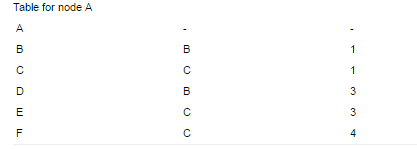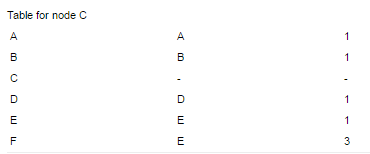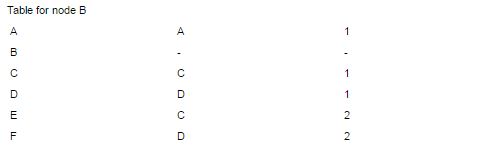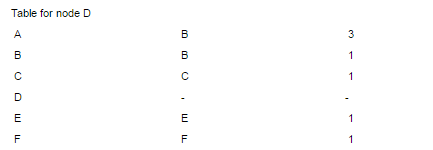Q. Continuing from the earlier problem, suppose at some time t, when the costs have stabilized, node A goes down. The cost from node F to node A at time (t + 100) is

Solution:
QUESTION: 15

The cyclomatic complexity of the flow graph of a program provides

Solution:
QUESTION: 16

What is the largest integer m such that every simple connected graph with n vertices and n edges contains at least m different spanning trees?

Solution:

A graph is connected iff all nodes can be traversed from each node. For a graph with n nodes, there will be n-1 minimum number of edges.
Given that there are n edges, that means a cycle is there in the graph.
The simplex graph with these conditions may be: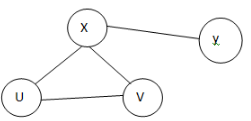Now we can make a different spanning tree by removing one edge from the cycle, one at a time.
Minimum cycle length can be 3, So, there must be atleast 3 spanning trees in any such Graph.

QUESTION: 17

For the undirected, weighted graph given below, which of the following sequences of edges represents a correct execution of Prim's algorithm to construct a Minimum Span­ning Tree?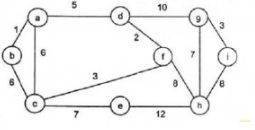Solution:

In prims algorithm we start with any node and keep on exploring minimum cost neighbors of nodes already covered.

QUESTION: 18

Consider a directed graph with n vertices and m edges such that all edges have same edge weights. Find the complexity of the best known algorithm to compute the minimum spanning tree of the graph?

Solution:

It’s a special case in which all edge weights are equal, so dfs would work and resultant depth first tree would be the spanning tree. So the answer would be O(m+n).

QUESTION: 19

You are given a graph containing n vertices and m edges and given that the graph doesn’t contain cycle of odd length. Time Complexity of the best known algorithm to find out whether the graph is bipartite or not is

Solution:

It is by definition that a graph is bipartite iff it does not contain odd length cycles. So the answer is O(1).

QUESTION: 20

Let G be a simple graph with 20 vertices and 8 components. If we delete a vertex in G, then number of components in G should lie between ____.

Solution:

Case 1: If the vertex we are deleting from G is an isolated vertex, which is a component by itself, then number of components in G becomes 7.
Case 2: If G is a start Graph, then by deleting the cut vertex of G, we get 19 components.
Hence, number of components in G should lie between 7 and 19.